Communications
Volume 3, Issue 5, September 2015, Pages: 86-92

Performance Evaluation of Cross Correlation Based Node Estimation Technique

Abu Sadat Md. Sayem, Md. Shamim Anower

Department of Electrical & Electronic Engineering, Rajshahi University of Engineering & Technology, Rajshahi, Bangladesh(A. S. Md. Sayem)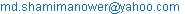(Md. S. Anower)

Abu Sadat Md. Sayem, Md. Shamim Anower. Performance Evaluation of Cross Correlation Based Node Estimation Technique. Communications. Vol. 3, No. 5, 2015, pp. 86-92. doi: 10.11648/j.com.20150305.12

Abstract: Estimating the number of operating nodes is an important factor in wireless communication network (WCN) in which the nodes are deployed in different forms to cover small or large areas of interest for a wide range of personal, scientific and commercial applications. It is important to estimate the number of operating nodes at any point in time for proper network operation and maintenance. Proper operation of a network depends on the total number of nodes present at a particular moment. Counting the number is very important in useful data collection, node localization and network maintenance. Also network performance depends on the area node ratio i.e. the number of operating nodes per unit area. So, node estimation is a vital requirement in wireless sensor network. At present, different estimation techniques exist but they are only effective for communication friendly networks. In underwater wireless sensor network node estimation faces a great difficulty due to underwater propagation characteristics such as high propagation delay, high absorption and dispersion. In such environment the number of nodes may vary frequently due to ad-hoc nature, power failure of nodes or environmental disaster. A statistical signal processing approach of node estimation is proposed in this paper and the performance of the proposed method is evaluated by comparing the results with other techniques. The nodes are considered as acoustic signal sources and their number is obtained through the cross correlation of the acoustic signals received at two sensors in the network. The mean of the cross correlation function is related with the number of nodes and is used as the estimation parameter in the process. Theoretical and simulation results are provided which show effectiveness of the signal processing approach instead of protocols in node estimation process.

Keywords: Wireless Communication Network (WCN), Cross Correlation Function (CCF), Estimation Parameter, Mean of Cross Correlation Function, Node Estimation, Underwater Acoustic Sensor Networks (UASN)

1. Introduction

A wireless communication network (WCN) is any type of  network that uses wireless data connections for connecting network nodes. WCNs may be classified by their geographical coverage area as: terrestrial (TWCN), space (SWCN), underground (UGWCN) and underwater (UWCN). Of these, the TWCN is the most dominant and covers almost the whole land area of the earth’s surface; for example, wireless mobile phone networks are widely used for personal communication and internet access. RFID systems have received much attention for applications such as monitoring and tracking objects. Besides TWCNs, SWCNs are another major application of WCN. The main goals of the SWCN are earth observation (EO), telecommunication with space vehicles and missions of localization from space. WSN technology can also be deployed underground where applications might be voice communication within underground environments (e.g., in caves or mines), or monitoring of soil conditions. In underwater wireless sensor networks operating nodes can communicate with each other for the purpose of environmental monitoring, seismic and acoustic monitoring to surveillance and national security, military and health care, discovering natural resources as well as extracting information for scientific analysis.

However, the number of operating nodes can vary with time due to various artificial as well as natural reasons (for example, some nodes might fail, some could be damaged, or batteries might fail). So, it is a matter of great interest for a communication network to know how many operating nodes or transmitters are available in the region at any point in time to ensure proper network operation (such as routing) as well as network maintenance (such as replacement of faulty nodes).

There have been many investigations regarding the estimation technique. For example, protocols [1-8] have been used to estimate the number of tag IDs in radio frequency identification (RFID) systems, which is a similar problem to the estimation of the number of nodes in wireless communication networks. Similarly, a Good-Turing estimator of node estimation for terrestrial sensor networks has been proposed in [9-11].

Although the abovementioned systems are easy to apply in RFID as well as terrestrial systems which are considered as communication friendly networks, they do not take into account the capture effect, which means that they are difficult to apply in underwater acoustic sensor networks (UASN). One solution has been proposed in [12,13], which proposed a node estimation technique taking the capture effect into account. The procedure is similar to probabilistic framed slotted ALOHA . Still it suffers from long propagation delays, high path loss in underwater acoustic network.

However, all of the abovementioned procedures for the estimation of the number of nodes in RFID systems and in wireless sensor networks are similar in that they are based on protocol design. But, underwater propagation characteristics  such as propagation delay, high absorption, and dispersion may make the use of protocol methods difficult. Using these conventional protocol-based techniques to obtain precise measurements is often expensive, inefficient and time consuming. Major challenges in the design of underwater acoustic networks are [15,16]:

&bull Battery power is limited and usually batteries can not be recharged, also because solar energy cannot be exploited;

&bull The available bandwidth is severely limited;

&bull Channel characteristics, including long and variable propagation delays, multi-path and fading problems;

&bull High bit error rates;

&bull Underwater sensors are prone to failures because of fouling, corrosion, etc.

In this paper, a simple estimation technique based on the cross correlation [17-20] of the acoustic signals received at two sensors in the network is proposed. In the proposed estimation technique the mean of CCF is used as the estimation parameter. The transmitted signals from a number of different random signal sources (nodes) within range are received by two sensors separated by a certain distance in the region; the received signals are summed at each of the two sensor locations, and these two signals are then cross-correlated. The estimate of the number of signal sources (assumed in our case the number of nodes in an underwater network) can be obtained based on the mean of the CCF. Finally, the performance of the proposed technique is compared with other techniques.

2. Theoretical Analysis of CCF

Let us, consider a 3D space where two receiving nodes are surrounded by N transmitting nodes as shown in Fig.1. Assuming that the transmitting nodes are the sources of white Gaussian signals and are uniformly distributed over the volume of a large sphere inside a cube, the centre of the sphere lays half way between the sensors, because only a sphere provides equal amounts of signals from every direction. The propagation velocity is constant, which is the proposed case, the sound velocity Sp, in the medium.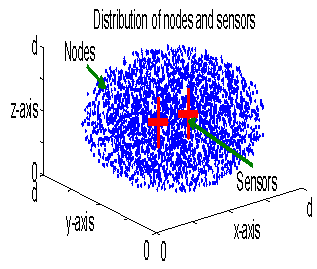Figure 1. Distribution of underwater nodes with N transmitting nodes.

Now, getting probe request, a node emits a very long Gaussian signal, which is recorded by the sensors with corresponding time delays. The signals in the sensors are cross-correlated, which takes the form of a delta function  as it is a cross correlation of two white Gaussian signals where one signal essentially is a delayed copy of the other. The position of this delta in the CCF will be the distance equal to the delay difference of the signals from the centre of the CCF where the position is called a bin in this paper. This holds for all nodes and the formation of CCF for N number of nodes can be expressed as follows :

If the transmitted signals from the nodes are denoted as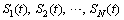respectively, the corresponding delays to reach the sensor 1 are denoted as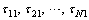, and the corresponding attenuations are as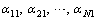, the composite signal at sensor 1 can be expressed as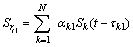(1)

Similarly, if the transmitted signals from the nodes are denoted asrespectively, the corresponding delays to reach the sensor 2 are denoted as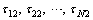, and the corresponding attenuations are as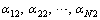, the composite signal at sensor 2 can be expressed as(2)

Assuming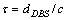is the time shift in cross correlation, and then the CCF is(3)

which takes the form of a series of delta functions as it is a cross correlation of two composite signals which are the summation of several white Gaussian signals.

One such obtained CCF with N (=1000) nodes is shown in Fig. 2.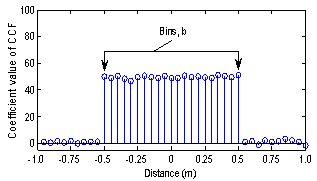Figure 2. Bins, b in the cross correlation process.

2.1. The Mean of the CCF

The mean of CCF is expressed by ensemble average of the signal cross correlation in  as(4)

where QT represents the acoustic power of the received signals from the nodes taken to be constant over time and space and ν, the creation rate of the random nodes whose unit is unit time per unit volume, Tr, total recording time,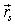, path length of node s from the origin,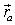, path length of first receiver from the origin, and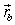,the path length of second receiver from the origin.

2.2. CCF as Binomial Distribution

The cross correlation technique can be reframed to a probability problem using the well-known occupancy problem which follows the binomial probability distribution from which a parameter is chosen to estimate the number of nodes of a network. Considering each delta function as a ball which occupies a bin according to the delay difference of corresponding recorded signals in the sensors, it is simple to model this cross correlation problem as a probability problem based on the well-known occupancy problem, i.e., the problem of placing N balls in b bins. It is known from  that the occupancy problem follows the binomial probability distribution in which the parameters are the number of balls i.e. nodes, N, and the inverse of the number of bins, b.

Occupancy problems deal with the pairings of objects and have a wide range of applications in different fields containing probabilistic and statistical properties. The basic occupancy problem is about placing m balls into b bins . If one threw some balls randomly towards several bins, the bins would be randomly filled by the balls, resulting in some bins being occupied by more than one ball, some by one while some may have none. In this work, the cross correlation process for node estimation is reframed as this occupancy problem. It describes the reframing process as follows.

&bull In this process to obtain a CCF, N nodes create N number of delta functions which occupy the place in the correlation length where the length is divided by b number of bins as shown in Fig. 2.

&bull Some bins are empty i.e. not occupied by any delta function; some are occupied by only one and others are more than one.

Moreover, the formation of cross correlation function to obtain node estimation satisfies the characteristics of binomial distribution as the number of trials i.e. the number of nodes is fixed, trials are independent in that sense the nodes are sending independent Gaussian signal, there exist only two possible outcomes, success or failure, for every trial which indicates that delta for a particular node is occupying a bin or not, each trial has the same probability of success which is one on the number of bins, b. As the cross correlation function follows the binomial distribution, its mean is easy to obtain which is discussed in the following section.

3. Estimation of the Number of Nodes, N

It is discussed in the previous section that the cross correlation function follows the binomial probability distribution in which the parameters are the number of balls i.e. nodes, N, and one on the number of bins, b. Then the expected value, i.e. the mean, m of the CCF is defined as :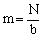(5)

where b is the number of bins in the cross correlation process and is obtained from the experimental setup with sampling rate, SR, distance between sensors, dDBS, and speed of propagation, Sp as: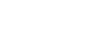(6)

Thus the estimation of N is obtained from (5) as: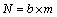(7)

This is the relationship between the number of nodes, N, and the mean, m, of the CCF. Since, we know b and can measure m from the CCF, we can readily determine the number of nodes, N.

4. Results and Discussion

Both theoretical and simulation results of the estimation of the number of nodes using this novel signal processing approach using cross correlation are provided in Fig. 3, Fig. 4 and Fig. 5. Simulations have been performed in the Matlab programming environment. These figures show that the theoretical and corresponding simulated results for the estimation of the number of nodes in a network in terms of the estimation parameter, m of CCF.

Above figures show that the simulations match the theory properly and is the indication of effectiveness of the process. The solid lines indicate the theoretical results and the circles the corresponding simulated results. The variations of b in the three different figures are as a result of varying dDBS (We consider sampling rate and propagation speed constant). The distances between the sensors are: 0.125m in Fig. 3, 0.25m in Fig. 4 and 0.5 m in Fig. 5. The other parameters are radius of the sphere is 2000m, N=1, 10, 20,…, 100, signal length is 106 samples, signal propagation speed is 1500m/s, and sampling rate SR = 180 kSa/s.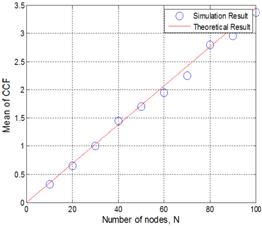Figure 3. Mean of CCF versus number of nodes, N for b=29, dDBS =0.125m.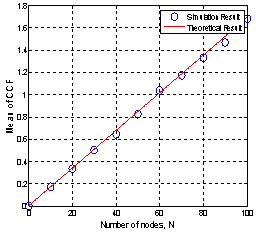Figure 4. Mean of CCF versus number of nodes, N for b=59, dDBS =0.25m.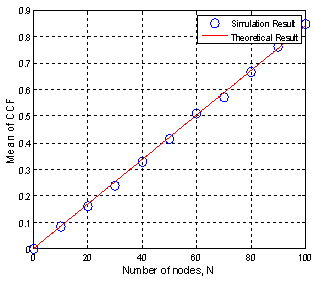Figure 5. Mean of CCF versus number of nodes, N for b=119, dDBS =0.5m.

The above mentioned results show that the simulated lines are very close to each other, which indicates that the process is good enough for estimation. At the same time, it is clear that the number of bins, b has an effect on the estimation parameter, which is depicted in the estimation expression (7). It can be seen that the value of the estimation parameter is lower in case of higher b and vice-versa and the simulated lines are more closer with the theoretical lines. It is also obvious from the results that a good approximation of the number of nodes, N, can be obtained from the m of the CCF even when the distances between sensors are small to place them in a single node.

Now, we will take another approach, the sampling rate will be doubled (360kSa/s) and the process will be repeated. A comparison will be observed for our estimation process for the same number of bins as before.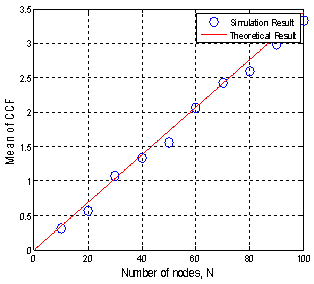Figure 6. Mean of CCF versus number of nodes, N for b=29 and dDBS =0.0625m.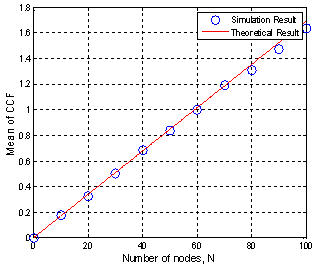Figure 7. Mean of CCF versus number of nodes, N for b=59 and dDBS =0.125m.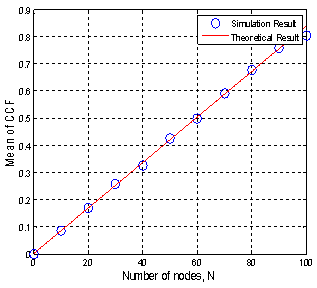Figure 8. Mean of CCF versus number of nodes, N for b=119 and dDBS =0.25m.

From Fig. 6 to Fig. 8 it can be observed that improvement in result occurs with the increase in number of bins as before.

From Fig. 3 to Fig. 8 it can be said that the result depends on the number of bins, b and variation in any parameter (s) in expression (6) affects the result.

Now, the result will be shown for the estimated number of nodes, N (estimated) with respect to exact number of nodes, N.

Fig. 9 shows the comparison of theoretical and simulated number of estimated nodes (for bin number 119). In this figure, the solid line indicates the theoretical result and the circles the corresponding simulated results. From Fig. 9, it can be seen that, the theoretical and simulated results are very close to each other, which signify the validity of the proposed approach.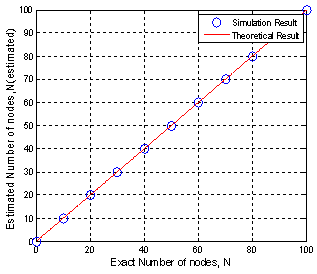Figure 9. Comparison of theoretical and simulated number of estimated nodes.

4.1. Analysis of Error in Estimation

Numerically, estimation error can be represented in different ways: such as- (i) as a true error which is the exact deviation of the estimated value from the true value, or (ii) as a statistical error which is obtained from several estimated values using the least squares technique. As the proposed cross correlation is a statistical technique, the statistical error, the coefficient of variation (CV), is used as its error in estimation in order to fully assess the accuracy of the proposed estimation techniques. To obtain a simulated CV of estimation, a simulation process is run 1000 times for a particular N and b. From these 1000 values of estimated N, the standard deviation and mean of estimation and, thus, the CV, are obtained. In this case firstly, the ratio (R) of standard deviation and mean of the CCF from 100 iterations, and then the estimated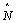using the expression of N related to this m, are obtained. Secondly, to obtain the CV, the same process is continued 1000 times without any change in parameters and the values of all estimatedare recorded. Finally, the CV for one iteration is obtained from the ratios of the standard deviation to the mean of those values as :Now, if we use iteration u, the standard deviation and, thus, the CV, are reduced to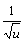so that the CV after the uthiteration isIt is noted that, if the number of nodes are increased, the standard deviation and mean of estimation increase by the same amount. Thus, the CV remains the same for all N, i.e., it is independent of N.

Now, the result will be shown graphically for bin number 19 and 99.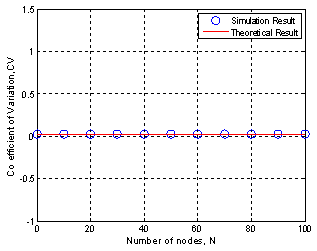Figure 10. CV versus N for b=19.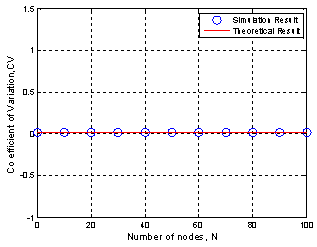Figure 11. CV versus N for b=99.

From Fig. 10 and Fig.11 it can be seen that CV is independent of the number of nodes.

4.2. Comparison of CV with Previous Estimation Techniques

Now, the proposed technique will be compared, in terms of error (CV), with those of conventional protocol-based techniques: the probabilistic framed slotted ALOHA (PFSA) , the Good-Turing (GT)  estimator protocol and DIIPUC  and two sensors cross correlation technique . The CVs are compared keeping the estimation time fixed.

In the above figure CCF: MEAN is the proposed method, CCF: RATIO is two sensors cross correlation technique  and rest three are conventional techniques.

In the above comparison it is considered a very long fixed signal length, Ns, of 158093 samples, Sampling rate 390000 HZ, signal propagation speed, 1500m/s, bin number119 and dDBS=0.25m. For conventional techniques the considered values of the parameters are: First frame size, F1=512; the maximum transmission range of the probing node is Rt = 2000m; the number of bits per packet is Bn= 112 bit/packet. The bit rate of the channel, BR= 15kbps considering 15kHz bandwidth and BPSK modulation technique, number of packets per slot, ρ =1for DIIPUC, ρ is 4 is GT, ρ is 1.59 for PFSA and estimation time is 40.5367 seconds.Figure 12. Comparison of CV of our proposed method with previous techniques.

Corresponding to CV it can be said that although Good Turing (GT)  method is better for fewer numbers of nodes the proposed technique is better than previous estimation techniques.

It can be seen that in the proposed estimation technique CV is dependent on the number of bins and that bin is proportional to the sampling rate (SR) and distance between sensors; CV is independent of the number of nodes. Thus, an error in estimation can be obtained as low as desired by increasing b (without exceeding the limit of the SR and dDBS).

5. Conclusion

In this paper cross correlation, a statistical signal processing approach for node estimation in underwater wireless sensor network has been presented. Here, the estimations are obtained using the statistical property of the cross correlation (of two composite signals) function. The proposed cross correlation technique is suitable for any environment networks with more accurate estimation than with the conventional techniques. Error in estimation of the number of nodes is investigated. The proposed method is compared with the conventional techniques with respect to error in estimation that demonstrate the superior performance of this technique to the previous methods. The paper includes an initial verification of the performance of the proposed techniques and suggests other issues such as energy and time requirement for estimation for future verification.

References

1. M. Kodialamand T. Nandagopal, "Fast and reliable estimation schemes in RFID systems," 12th annual international conference on Mobile computing and networking (MobiCom'06), Los Angeles, CA, USA, ACM.2006.
2. M.A. Bonuccelliand F. Lonetti, et al., "Tree Slotted Aloha: a New Protocol for Tag Identification in RFID Networks," Proceedings of the 2006 International Symposium on World of Wireless, Mobile and Multimedia Networks, IEEE Computer Society.2006.
3. M. A.-I. Center, Draft protocol specification for a 900 MHz class 0 radio frequency identification tag, http://www.epcglobalinc.org, Feb. 2003.
4. C. Lawand K. Lee, et al., "Efficient memory less protocol for tag identification (extended abstract),"Proceedings of the 4th international workshop on Discrete algorithms and methods for mobile computing and communications", Boston, Massachusetts, USA, ACM: 75-84.2000.
5. J. Myung,W. Lee and J. Srivastava, "Adaptive binary splitting for efficient RFID tag anti-collision," IEEE Communications Letters, vol. 10 (3), pp.144–146, Mar. 2006.
6. J. Myung and W. Lee, et al., "Adaptive binary splitting for efficient RFID tag anti-collision," IEEE Communications Letters 10(3): 144-146.2006.
7. J. Myung and L. Wonjun, et al., "Tag-Splitting: Adaptive Collision Arbitration Protocols for RFID Tag Identification," IEEE Transactions on Parallel and Distributed Systems 18: 763-775.2007.
8. M.A. Bonuccelli, F. Lonetti and F. Martelli, "Perfect tag identification protocol in RFID networks,"http://arxiv.org/PS_cache/arxiv/pdf/0805/0805.1877v1.pdf, May 13, 2008.
9. C. Budianu, S. Ben-David and L. Tong, "Estimation of the number of operating sensors in large-scale sensor network with mobile access," IEEE Transactions on Signal Processing, vol. 54, no. 5, pp. 1703–1715, May 2006. doi: 10.1109/TSP.2006.871973.
10. C. Budianu and L. Tong, "Estimation of the number of the operating sensors in a sensor network," presented at 2003 Asilomar Conf. Signals, Systems, Computers, Monterey, California.
11. C. Budianu and L. Tong, "Good-Turing estimation of the number of operating sensors: a large deviations analysis," Int. Conf. Acoustics, Speech, Signal Processing (ICASSP), Montreal, QC, Canada, May 2004, vol. 2, pp. 1029–1032. doi: 10.1109/ICASSP.2004.1326436.
12. M. S. A. Howlader M. R. Frater, et el.,"Estimating the Number and Distribution of the Neighbors in an Underwater Communication Network," Second International Conference on Sensor Technologies and Applications (SENSORCOMM'08).2008.
13. M. S. A. Howlader and M. R. Frater, et al., "Estimation in underwater sensor network taking into account capture," IEEE OCEANS '07, Aberdeen, Scotland.2007.
14. L. Liu, S. Zhou and C. Jun-Hong, "Prospects and problems of wireless communication for underwater sensor networks," Wireless Communication Mobile Computer 2008, Published online in Wiley InterScience. DOI=http://doi.wiley.com/10.1002/wcm.654.
15.  J.H. Cui, J. Kong, M. Gerla and S. Zhou, "Challenges: Building scalable mobile underwater wireless sensor networks for aquatic applications," IEEE Network, Special Issue on Wireless Sensor Networking, pp. 12-18, 2006.
16. I. F. Akyildiz, D. Pompili and T. Melodia, "Underwater acoustic sensor networks: Research challenges, Ad Hoc Networks," pp. 257–279, 2005.
17. P. Roux, K. Sabra, W. Kuperman and A. Roux, "Ambient noise cross correlation in free space: theoretical approach," J. Acoustic Soc. Am., 117, 79–84, 2005.
18. R. Snieder, "Extracting the Green’s function of attenuating heterogeneous acoustic media from uncorrelated waves," J. Acoustic Soc. Am., Vol. 121, No. 5, 2637-2643, May 2007.
19. O.A. Godin, "Recovering the Acoustic Green’s function from ambient noise cross correlation in an inhomogeneous moving medium," Physical Review Letters, The American Physical Society, 97, 054301(2006).
20. K.G. Sabra, P. Roux and W.A. Kuperman, "Emergence rate of the time-domain Green’s function from the ambient noise cross - correlation function," J. Acoustic Soc. Am., Vol. 118, No. 6, 3524–3531, December 2005.
21. M. S. Anower, M. A. Motin, A. S. M. Sayem, and S. A. H. Chowdhury, "A node estimation technique in underwater wireless sensor network," In Proceedings of International Conference on Informatics, Electronics & Vision (ICIEV), 17–18 May, 2013, pp. 1–6. doi: 10.1109/ICIEV.2013.6572582.
22. H.Vogt, "Efficient object identification with passive RFID tags", Lecture Notes in Computer Science 2414.2002.
23. W. Feller, "An Introduction to Probability Theory and its Applications," John Wiley, 1968.
24. M. S. Anower, S. A. H. Chowdhury, Jishan-E-Giti, A. S. M. Sayem and M.I. Haque "Underwater network size estimation using cross - correlation: selection of estimation parameter," Proceedings of the 9th International Forum on Strategic Technology (IFOST-2014), 21-23 October-2014 in Cox’s Bazar, Bangladesh. DOI:10.1109/IFOST.2014.6991097.
25. M. S. Anower, "Estimation using cross - correlation in a communications network," Ph.D. dissertation, SEIT, University of New South Wales at Australian Defense Force Academy, Canberra, 2011.

 Contents 1. 2. 2.1. 2.2. 3. 4. 4.1. 4.2. 5.
Article ToolsAbstractPDF(671K)